# Using Foundry to Explore Upgradeable Contracts (Part 1)

Posted on December 19th, 2022 by David Kretzmer
Posted in[Prerequisites: Basic knowledge of Solidity]

Making contracts upgradeable offers a lot of flexibility, but also makes the code more difficult to reason about. This is mostly due to the need to circumvent Solidity’s type system, which means that the compiler’s ability to catch mistakes is severely limited. The recent Audius attack is a good example of how easy it is to make a mistake when using upgradeable contracts, and underlines the importance of really understanding what is going on underneath the abstractions.

This post is the first in a two-part series: We will see how upgradeable contracts can be implemented and, in particular, what can go wrong. The second part will then take a closer look at the Audius attack, recreating each step performed by the attacker. Even with the knowledge of the first part, the bug that was exploited by the attacker remains hard to spot if you are not looking for it.

Throughout the post, we use Foundry tests written in Solidity to illustrate the various techniques used to make contracts upgradeable, and to make experimentation and exploration as easy as possible. All code is available in this repo.

A word of caution: None of the code presented here should be used in production, as it was not developed with this purpose in mind.

## Preliminaries

The immutability of the blockchain clashes fundamentally with the traditional software development process, where code is constantly updated to fix bugs and add new features. But even though the code for a particular smart contract remains immutable, there are still techniques available that have the same effect as updating the code.

This is made possible primarily through a combination of two features: The `delegatecall` EVM instruction and fallback functions in Solidity.

## Code and Storage

To understand `delegatecall`, it is helpful to picture the state of the EVM during the execution of a smart contract: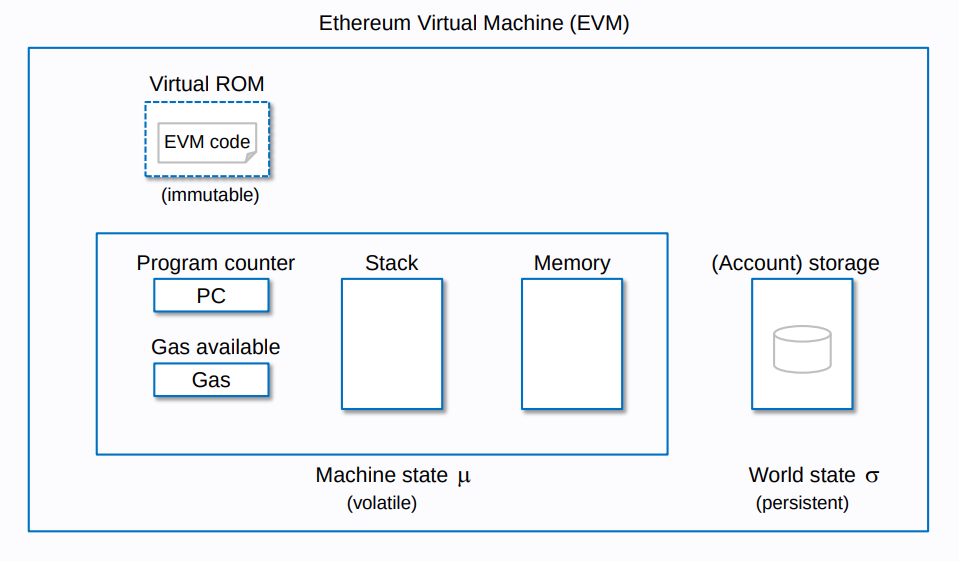We can distinguish between persistent and volatile execution state. Persistent state is remembered across transactions, while volatile state is immediately forgotten after a transaction has been executed. Both the EVM code (smart contract code) and storage are persistent, while stack, memory, program counter and remaining gas are volatile. Here, we are mostly interested in the code and storage components, as they are the most important in order to understand upgradeable contracts.

While both code and storage are persistent, there is a fundamental difference between the two: Storage is mutable and can be modified, while code is immutable once deployed. To this end, the code is kept separate from the storage in a read-only part of the memory. (This is different from a typical von-Neumann architecture like x86, where code and memory share the same address space.) This separation makes the delegatecall instruction possible, which uses the code from one contract and the storage from another (see next section).

It is important to distinguish between storage and memory. Storage is persistent and maps 32 byte addresses to 32 byte values, which are known as slots. On the other hand, memory is volatile and maps 32 byte addresses to 1 byte values. In other words, storage is word-addressable (where a word is 32 bytes), while memory is byte-addressable.

In Solidity, any variable declared at the contract level is mapped to one or more storage slots. For example, consider the following contract:

``````contract Token {
uint256 public totalSupply;

constructor() {
owner = msg.sender;
}

function mint(address user, uint256 amount) external {
require(msg.sender == owner, "Only owner is allowed to mint");
balanceOf[user] += amount;
}
}``````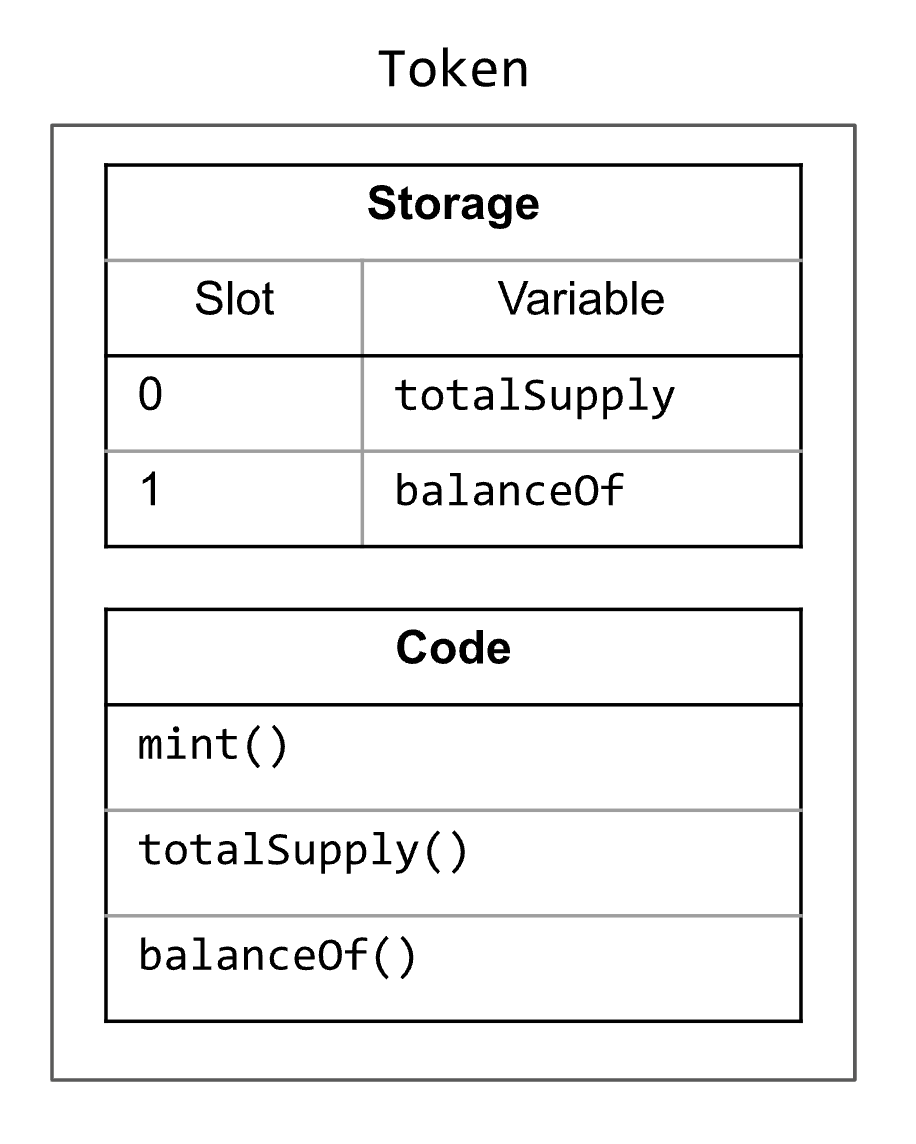The first variable is mapped to slot 0, the second to slot 1, etc1. Schematically, we can represent this contract as shown on the right. (Note that `Token::owner` is immutable and thus does not occupy any storage slot.) While values of simple types like `address` and `uint256` require at most 32 bytes and thus fit into a single storage slot, this is not the case for mappings and dynamic arrays. For this reason, even though `balanceOf` is mapped to slot 1, nothing is actually stored in this slot.Instead, if we want to access `balanceOf[addr]`, the corresponding slot is computed as follows:

``````keccak(
)``````

We take the key (here: `addr`) and the slot number of the mapping (here: 1), zero-extend them both to 32 bytes, concatenate them (denoted by ++), and finally compute the keccak hash of the result. The following Foundry test shows how this can be expressed in Solidity:

``````Token t = new Token();
t.mint(Alice, 5 ether);

bytes32 aliceBalanceSlot = keccak256(
abi.encodePacked(uint256(uint160(Alice)), uint256(1))
);

// Check that we have correctly computed the slot at which Alice’s balance is stored
assertEq(aliceBalance, t.balanceOf(Alice));``````

In this example, we want to retrieve the value of `t.balanceOf(Alice)`, but instead of doing so directly we instead manually compute the slot at which Alice’s balance is stored. To this end, `aliceBalanceSlot` is computed following the description above. We then use the Foundry-provided cheat code vm.load() to load the value stored at the computed slot in contract `t`. Finally, we use `assertEq()` to ensure that we have actually loaded the correct value. See Storage.t.sol
for a full example.

For a simple contract like Token we can easily compute the slots for contract variables manually. However, for more complex contracts that use inheritance, or that have multiple variables that are stored in the same slot, this task becomes more difficult. Fortunately, Foundry provides a command to visualize the storage layout of a contract. For example, to display the storage layout of the Token contract, you can use the following command:

``````\$ forge inspect Token storage-layout –-pretty
``````

This works for any contract that is part of the current Foundry project. If you want to analyze already deployed contracts, have a look at the sol2uml tool.

## `delegatecall`

If code is immutable, how is it possible to upgrade smart contracts and change their behavior? This is primarily due to the `delegatecall` instruction2, which uses the code of one contract and executes it using the storage of another contract. This can be illustrated by a simple example:

``````contract Counter {
uint256 number;

function get() external view returns(uint256) {
return number;
}

require(n <= 5, "Max increment is 5");
number += n;
}
}``````
``````contract DelegateCounter {
uint256 number;

function get() external view returns(uint256) {
return number;
}

function delegateAdd(Counter c, uint256 n) external {
bytes memory callData = abi.encodeWithSignature("add(uint256)", n);

if(!ok) revert("Delegate call failed");
}
}``````

The Counter contract represents a counter that can only increase by at most five steps at a time. To this end, it defines a function `add()` that enforces this requirement, and a function `get()` to retrieve the current counter value. The `DelegateCounter` contract is essentially the same as `Counter`, except for the function `delegateAdd()`. To explain how `delegateAdd()` works, it is helpful to visualize both contracts: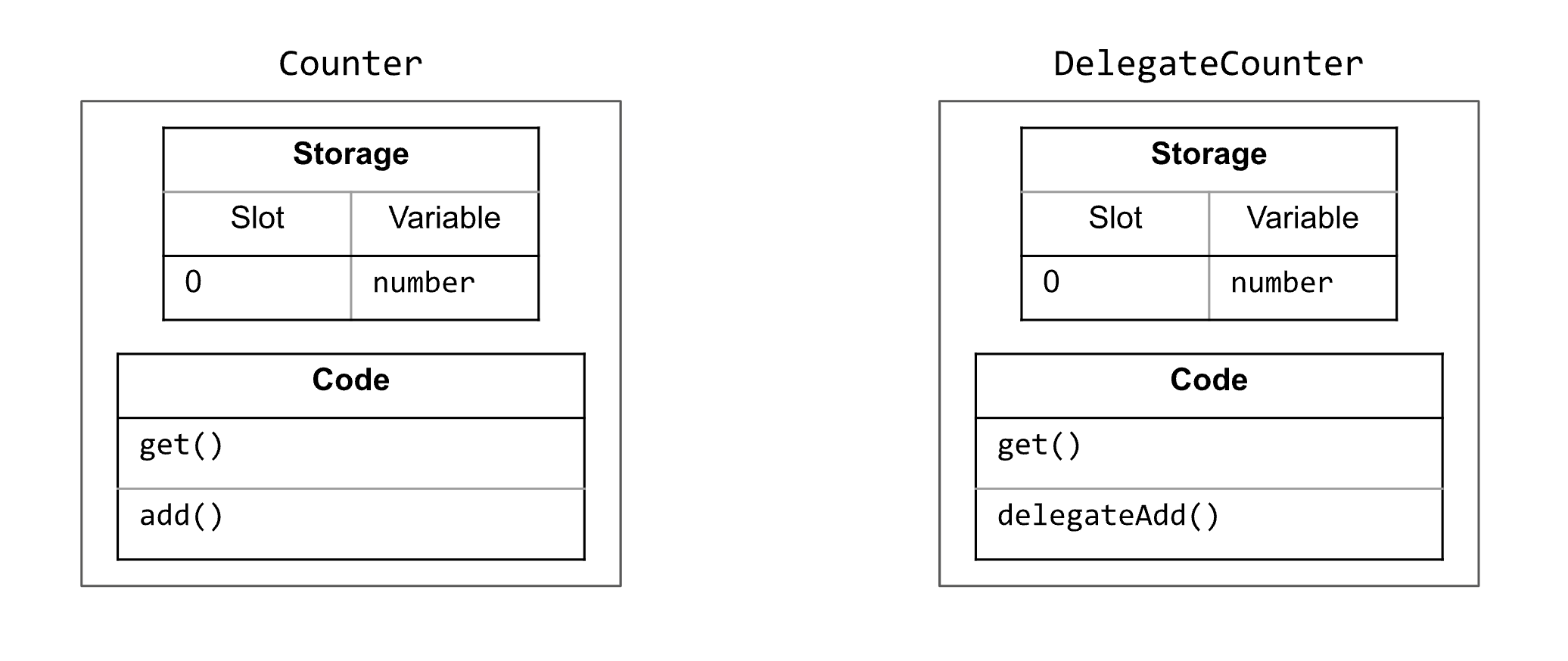Intuitively, `delegateAdd()` uses `delegatecall` to execute the function `add()` from contract `Counter` using the storage from `DelegateCounter`. For this to work, both contracts should have compatible storage layouts, meaning they should assign the same variables to the same storage slots.

`delegatecall` is a low-level primitive in Solidity and less convenient to use than normal function calls. In general, whenever we want to call a function on a contract, we need to specify both which function we want to call and which arguments we want to pass. This information needs to be encoded in a well-known format so that the target contract knows how to interpret it. This format is also known as the Application Binary Interface (ABI) and is described in the `Contract ABI Specification`. For normal function calls, Solidity does this encoding for us, but when using `delegatecall` we need to do it ourselves. This is done in the first line of `delegateAdd()`:

``bytes memory callData = abi.encodeWithSignature("add(uint256)", n);``

The first argument of `encodeWithSignature()` denotes the signature of the function we want to call, and the remaining arguments denote the values we want to pass to that function. In the above example, we encode a call to a function named add that takes a single argument of type `uint256`, whose value should be `n`. If we assume that `n` is, for example, 4, then `callData` will look as follows:

````0x1003e2d20000000000000000000000000000000000000000000000000000000000000004`
```

(You can verify this by adding `console.logBytes(callData)` to the `delegateAdd()` function.)

The first four bytes (blue part) represents the `function selector`, which is computed by taking the four most significant bytes of the keccak hash of the function signature. The function signature is “add(uint256)”, and we can use the cast command-line utility that comes with Foundry to compute its keccak hash:

``````\$ cast keccak "add(uint256)"
0x1003e2d21e48445eba32f76cea1db2f704e754da30edaf8608ddc0f67abca5d0```
```

As you can see, the highlighted four bytes of the hash match the most significant four bytes of `callData`.

The function selector is followed by the argument (the red part of the value of `callData`), which is simply the value 4 represented as a `uint256`, i.e., as a 32 byte unsigned number.

Now that we have stored the encoded function call in `callData`, we can pass it to `delegatecall`:

````(bool ok,) = address(c).delegatecall(callData);`
```

This line executes the function `Counter.add()` in the context of the current contract. In particular, any storage access that is performed by `Counter.add()` will use the storage of the calling contract, which in this case is of type `DelegateCounter`. Thus, when the `Counter.add()` function writes to slot 0 in order to update the storage variable `number`, it updates the storage of `DelegateCounter` and not that of `Counter`.

`delegatecall` returns two values: A boolean to indicate whether the call succeeded, and a byte array containing any returned data. Since `Counter.add()` does not return anything, `delegateAdd()` ignores the return data and only checks whether the call was successful. This is particularly important because when using `delegatecall`, reverts in the called function are not automatically propagated3:

``if(!ok) revert("Delegate call failed");``

To make all this a bit more concrete, here is an example:

``````Counter c = new Counter();
DelegateCounter d = new DelegateCounter();

// Sanity check: both counters should start at zero
assert(c.get() == 0);
assert(d.get() == 0);

// Check that `d` has been updated and that `c` remains unchanged
assert(c.get() == 0);
assert(d.get() == 4);``````

We first create new instances of the `Counter` and `DelegateCounter` contracts and convince ourselves that they both start at zero. Then comes the interesting part, namely the call `d.delegateAdd(c, 4)`. As explained above, `delegateAdd()` essentially calls `c.add(4)` in such a way that all storage accesses refer to `d` and not to `c`. This is verified by the following two asserts, which check that `c` is still zero, while `d` has been updated.

Now it may become clear how `delegatecall` is used to implement upgradeable contracts, because we can pass any contract to `delegateAdd()` that implements a function with signature `add(uint256)`. Thus, even though the `DelegateCounter` remains immutable, we can change its behavior by passing some other contract to `delegateAdd()`. However, to fully implement upgradeable contracts we need to look at one more feature, namely fallback function. This is covered in the section Fallback functions. However, before we move on, it is useful to see how to handle the second return value of `delegatecall`, i.e., the byte array containing the data returned from the called function.

## Handling return values

As we have already noticed, using `delegatecall` is much less convenient than normal function calls because we have to manually encode the call according to the ABI. The same is true for the data returned from the call: We simply get a raw byte array that we need to decode ourselves according to the return type of the function that was called. To illustrate how to do this, we now implement a `delegateGet()` function for `DelegateCounter`:

``````contract DelegateCounter {
// ...

function delegateGet(Counter c) external returns(uint256) {
bytes memory callData = abi.encodeWithSignature("get()");
(bool ok, bytes memory retVal) = address(c).delegatecall(callData);

if(!ok) revert("Delegate call failed");

return abi.decode(retVal, (uint256));
}
}``````

The implementation is very similar to `delegateAdd()`: We first ABI-encode the call we want to perform and then use `delegatecall` to make the call. However, this time we also handle the data returned by the call, which we store in `retVal`. Since `get()` returns a uint256, and the ABI specifies that values of fixed-width types like uint256 are encoded by simply taking their big-endian representation and padding the result to 32 bytes, the return data can be decoded by simply casting retVal to uint256:

``return uint256(bytes32(retVal));``

However, for complex types decoding becomes more involved. Fortunately, Solidity provides the function `abi.decode()` that can perform the decoding for us. Using this function, we can rewrite the return statement as follows:

``return abi.decode(retVal, (uint256));``

The function `abi.decode()` takes two arguments: A byte array containing some ABI-encoded values, and a tuple with the types of the encoded values.

## Generalization

In preparation for later, we can make one final change to `delegateGet()` in order to generalize the way the return data is handled. Note that when we decode the return data with `abi.decode(retVal, (uint256))`, we are hardcoding the return type. If we want to be able to use `delegatecall` with arbitrary functions, then we also need to be able to handle arbitrary return data. This is not possible in pure Solidity, so we need to turn to assembly. In particular, we need to replace

``return abi.decode(retVal, (uint256));``

with

``````assembly {
return(data, size)
}``````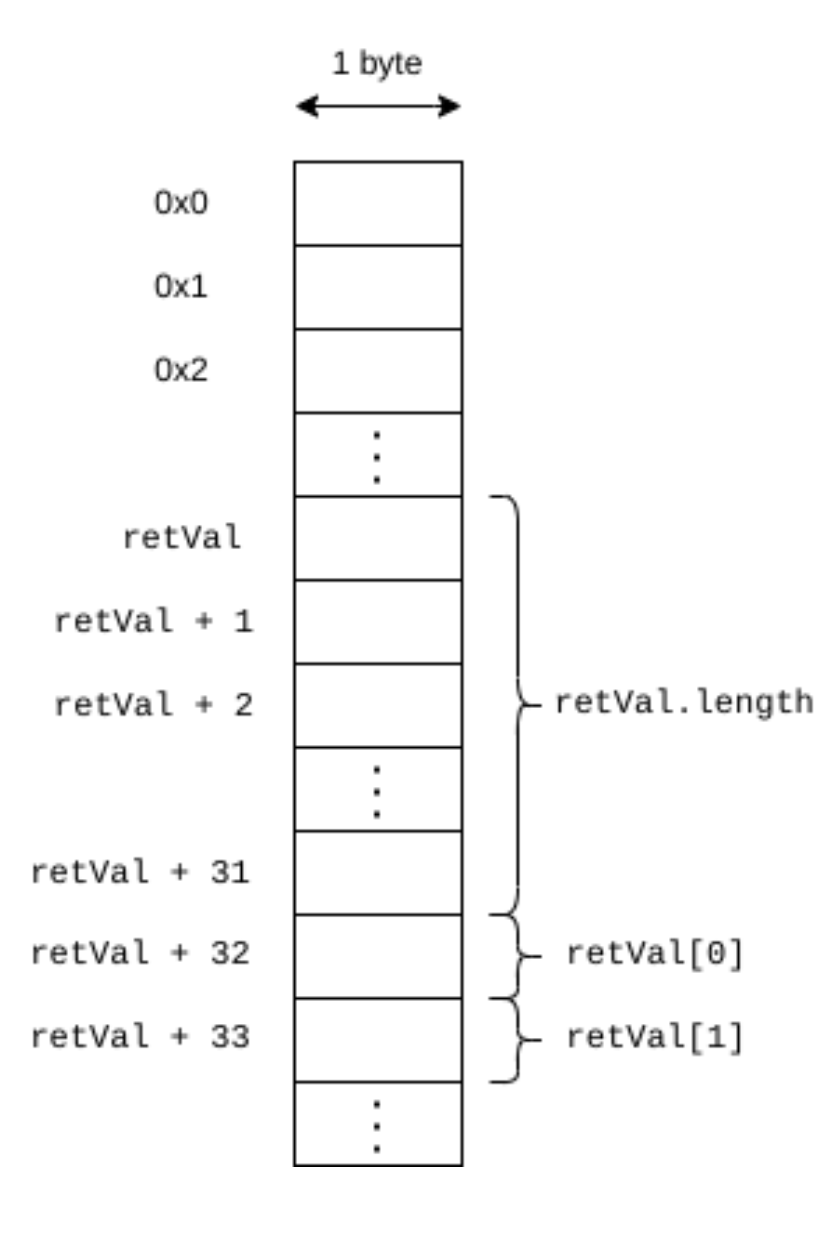The `return(data,size)` instruction ends execution of the current function and returns the data in the memory range given by `data` and `size`, where data denotes the starting address and `size` denotes the size of the data in bytes (see the Yul specification for more details). In the above example, the way `data` and `size` are computed may not be obvious. To understand this, it is important to know how arrays are laid out in memory. First, note that when we refer to a memory variable like `retVal` from an assembly block, we are actually referring to its address. Thus, when we use `retVal` in the above assembly block, we are referring to the address in memory at which the byte array denoted by `retVal` starts. Second, Solidity lays out arrays in memory as follows4: First comes the array length, stored as a 32 byte unsigned number, and then come all the array elements. Thus, the array length of `retVal` is stored directly at the address of `retVal` (which we load via `mload`), and to get the address of the array elements we need to add a 32 byte offset to `retVal`.

With the above assembly, we can simply forward any return data from `delegatecall`, without needing to know the type of the encoded value. This allows us to call arbitrary functions without knowing their return types in advance.

To play around with the code have a look at DelegateCall.t.

## Fallback functions

Fallback functions are another useful feature when implementing upgradeable contracts. They allow the developer to specify what should happen when a non-existent function is called. The default behavior is to revert, but this may be changed.

``````interface Ifc {
function hello() external;
function bye() external;
}``````
``````contract C {
event Log(string msg);

function hello() external {
emit Log("hello");
}

fallback() external {
emit Log("fallback");
}
}``````

Above we define a simple interface with functions `hello()` and `bye()`. In addition, we define a contract `C` that contains a function `hello()` and a fallback function. Now consider the following example:

``````Ifc ifc = Ifc(address(new C()));
ifc.hello(); // Emits Log("hello")
ifc.bye();   // Emits Log("fallback")``````

We create a new instance of contract `C` and cast it to `Ifc`, which allows us to call both `hello()` and `bye()`. When we call `bye()`, which is not defined by `C`, the fallback function is executed instead.

One useful fact is that we can use `msg.data` to access the original call data that triggered the fallback function. For example, if you add `console.logBytes(msg.data)` to the fallback function of `C`, then the following log message is produced when calling `ifc.bye()`:

``0xe71b8b93``

As you would expect, this is simply the function selector for `bye()` (since `bye()` has no parameters, there are no encoded arguments). This means that by inspecting `msg.data` we can determine which function the user originally intended to call.

See Fallback.t.sol
for the full example.

Using both `delegatecall` and fallback functions we can implement a general solution for upgradeable contracts based on proxies. The core idea is as follows: For each contract whose code we want to be upgradeable, we actually deploy two contracts: A proxy contract and a logic contract. The proxy is the contract that stores all the data, and the logic contract contains the functions that operate on that data. Users will only interact with the proxy contract. When a user calls a function on the proxy, the proxy forwards the call to the logic contract using a delegate call. Because the proxy uses a delegate call, executing the function from the logic contract affects the storage of the proxy. Thus, when using upgradeable contracts, the proxy holds the state, while the logic contract holds the code. From the point of view of the user, the proxy behaves the same as the logic contract. Upgrading the contract simply means that the proxy is using a new logic contract.

## A First Attempt (Does Not work)

By the above explanation, one could maybe be tempted to implement the proxy contract as follows:

``````contract FaultyProxy {

implementation = newImpl;
}

fallback() external payable {
(bool ok, bytes memory returnData) = implementation.delegatecall(msg.data);

if(!ok)
revert("Calling logic contract failed");

// Forward the return value
assembly {
return(data, size)
}
}
}``````

As its name suggests, this proxy does not work in general. However, it is still instructive to understand why it does not work, especially because the bug in the Audius protocol that we are going to look at is very similar to the one in the proxy above.

The proxy has a single storage variable, `implementation`, which stores the address of the logic contract. By calling `upgradeTo()` the logic contract can be changed, making the logic (in other words: the code) upgradeable. (Right now, anybody can call `upgradeTo()`, which is of course not desirable. We will come back to this later.) The final piece of the puzzle is the fallback function. Its purpose is to forward any call to the logic contract using `delegatecall`. (Except calls to `upgradeTo()` and `implementation()`, which are handled by the proxy itself.) But how do we know which function the user wanted to call? Fortunately, the original calldata that triggered the fallback function is accessible via `msg.data`5. As the calldata contains both the function signature and the argument values, we can simply pass `msg.data` to `delegatecall`. Afterwards, we check whether the call was successful. If not, we revert, otherwise we forward the return data.

The following example shows how the proxy is supposed to be used:

``````// (1) Create logic contract
Counter logic = new Counter();

// (2) Create proxy and tell it which logic contract to use
FaultyProxy proxy = new FaultyProxy();

// (3) To be able to call functions from the logic contract, we need to
//     cast the proxy to the right type

// (4) Now we treat the proxy as if it were the logic contract

// (5) Did it work? (Spoiler: no!)
console.log(“counter =”, proxied.get()); // Reverts!``````

The first two steps create the logic and the proxy contract, respectively. The second step also calls `upgradeTo()` so that the proxy knows which logic contract to use. The third step is needed to tell the Solidity compiler that we now plan to use the proxy as if it were the logic contract. The fourth step is where it gets interesting: We call the `add()` function on the proxy. Since the proxy does not define any function of that name, its callback function is executed. Inside the fallback function, `msg.data` contains the following call data:

``0x1003e2d20000000000000000000000000000000000000000000000000000000000000002``

This represents a call to a function with signature “add(uint256)” and argument 2 (see section delegatecall for how the call data is encoded). The fallback function then performs a `delegatecall` with the above calldata, executing the `add()` function from the `Counter` contract using the storage of the proxy.

Finally, in the fifth step, we try to retrieve the current counter value from the proxy. However, executing `proxied.get()` actually reverts! The cause for this error can easily be explained by visualizing both the proxy and the logic contract: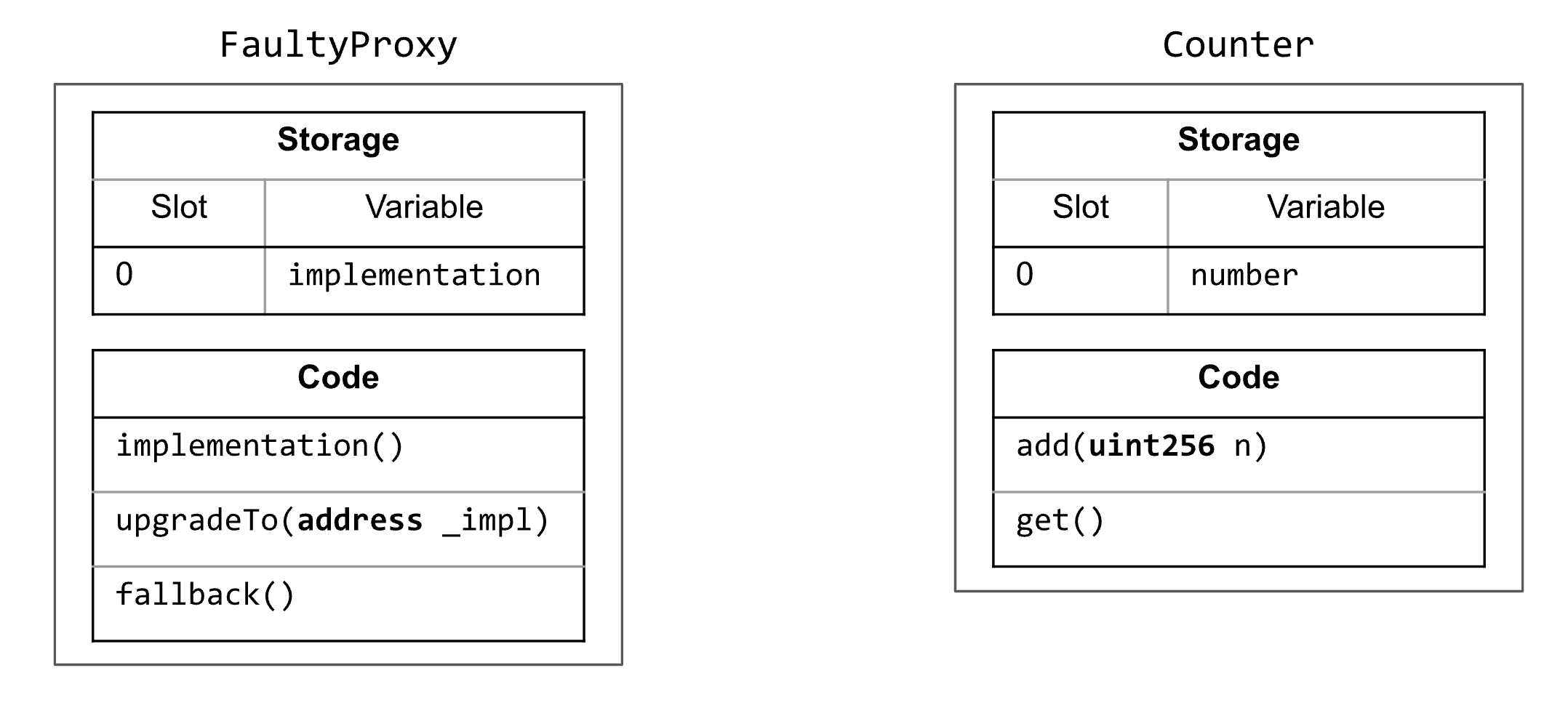When comparing the storage layouts of both contracts, one can notice that they store different variables in slot 0. This has an unfortunate consequence: When `Counter.add()` is executed using the storage of `FaultyProxy`, it modifies storage slot 0 in order to update `number`. However, in contract `FaultyProxy`, slot zero contains the value of `implementation`. Thus, when we call `proxied.add(2)` in step (4), we actually increase the address stored in `implementation` by two, making the address invalid. More precisely, the resulting address now points to an account to which, in all likelihood, no contract has been deployed. When making a delegate to an empty account, the call will succeed, but no data will be returned. However, since we do expect that a value of type `uint256` is returned, the test reverts.

See FaultyProxy.t.sol
for the code.

## A Working Solution (With Flaws)

How can we fix the storage slot collision between proxy and logic contract? A simple way would be to add a dummy storage variable before `number` in the `Counter` contract. Then, `number` would be stored in slot 1 and it would not clash with `implementation` from `FaultyProxy` anymore. However, this is not a great solution: It is brittle, can easily be forgotten, and may be difficult to enforce if the logic contract inherits from other contracts.

So how can the proxy contract store the address of the logic contract without creating a slot collision? There are multiple ways to go about it, see section Design Choices. Here, we will follow the Unstructured Storage Pattern, which is widely used (for example by OpenZeppelin, also see Upgradeability using Unstructured Storage) and provides a solution to this problem that does not require any changes to the logic contract. The idea is to let the proxy store the logic contract address in some far-away slot in such a way that the chances of a slot collision are negligible. With this idea we can implement a new proxy.

``````// This proxy is working but still has flaws, so don’t use it for anything serious
contract Proxy {
bytes32 constant IMPLEMENTATION_SLOT =
bytes32(uint256(keccak256('eip1967.proxy.implementation')) - 1);

bytes32 slot = IMPLEMENTATION_SLOT;
assembly {
sstore(slot, newImpl)
}
}

function implementation() public view returns(address impl) {
bytes32 slot = IMPLEMENTATION_SLOT;
assembly {
}
}

fallback() external payable {
(bool ok, bytes memory returnData) =
implementation().delegatecall(msg.data);

if(!ok)
revert("Calling logic contract failed");

// Forward the return value
assembly {
return(data, size)
}
}
}``````

The crucial difference between `Proxy` and `FaultyProxy` is that `Proxy` does not declare any storage variables. Instead, the address of the logic contract is stored in slot `IMPLEMENTATION_SLOT`, which is computed as the keccak hash of the string “eip1967.proxy.implementation” subtracted by one6. As the name suggests, this slot number was standardized in EIP-1967. Having a well-defined slot at which the logic contract is stored allows services like Etherscan to automatically detect if a contract functions as a proxy, in which case information about both the proxy and the logic contract can be shown. For example, if you look at the code of USDC on Etherscan, in addition to the normal “Read/Write Contract” tabs there are also options for “Read/Write as Proxy”, which provide a link to the current logic contract.

To upgrade the contract, the `upgradeTo()` function needs to modify the address at the slot given by `IMPLEMENTATION_SLOT`, which is possible using the `sstore` instruction. Note that we need to copy `IMPLEMENTATION_SLOT` to a local variable because it is not possible to read a constant from assembly directly. The function `implementation()` is implemented in a similar way to read the address stored at slot `IMPLEMENTATION_SLOT`. Finally, the fallback function remains unchanged, except that we now use the `implementation()` function instead of storage variable to get the logic contract address.

The fact that we use `sstore`/`sload` to access the logic contract instead of using a contract variable makes this proxy unstructured, which explains the name Unstructured Storage Pattern. We can again visualize both the proxy and the logic contract: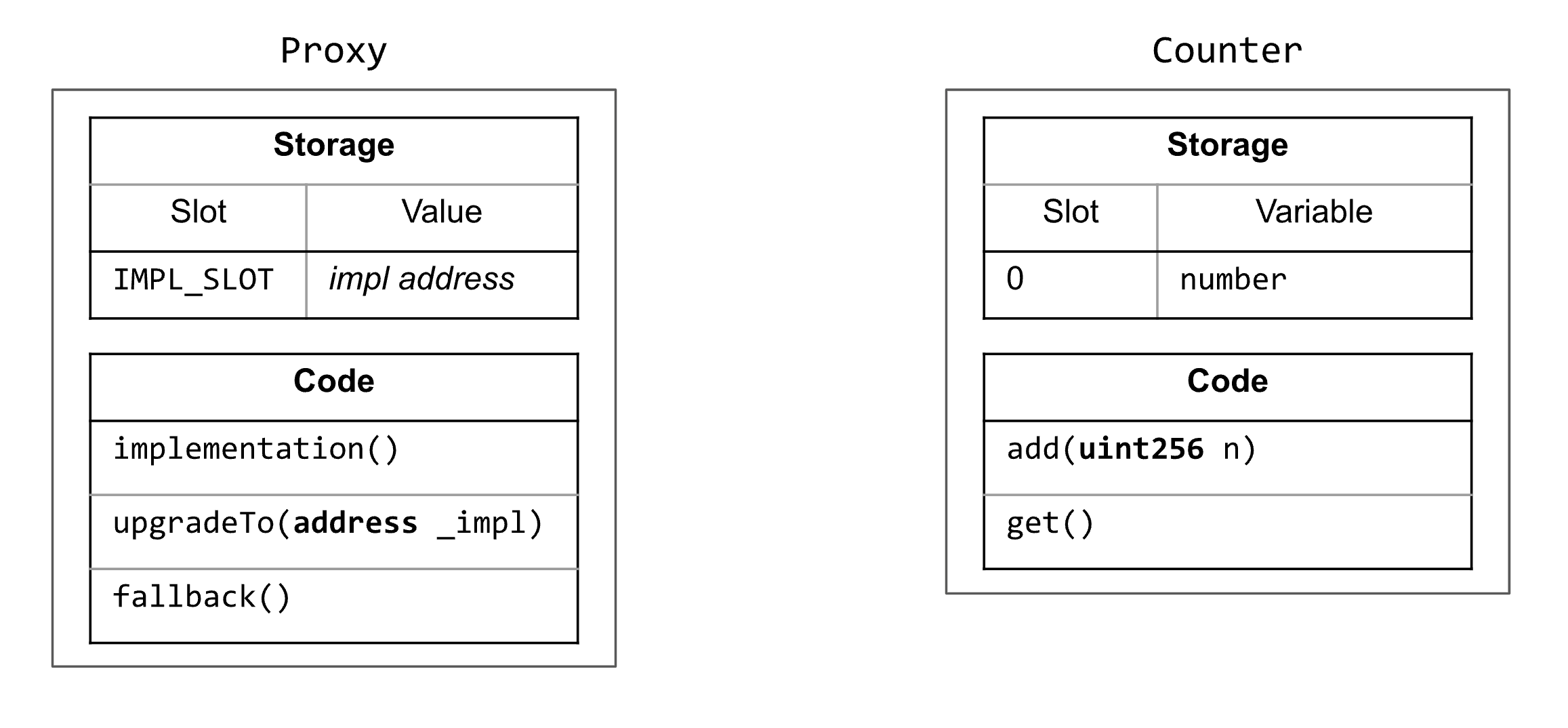(Here, `IMPL_SLOT = IMPLEMENTATION_SLOT`.) Previously, it was mentioned that when using `delegatecall` it must be ensured that both the caller contract and the callee contract have compatible storage layouts to prevent the callee from messing up the storage of the caller. For the `Counter` and `DelegateCounter` contracts from section delegatecall) this was easy to verify, since both contracts define exactly the same storage variables. On the other hand, `Proxy` does not have the same storage layout as `Counter`, but since both contracts use entirely different storage slots and thus do not step on each other’s toes, this is not a problem. (In fact, `Proxy` is completely independent of the concrete logic contract that is used, which is great because this means one only needs to write a single proxy that can be used by everyone.)

Of course, this is only safe if the slot denoted by `IMPLEMENTATION_SLOT` does not accidentally clash with any storage variable from the logic contract. Is this actually guaranteed? First, note that `IMPLEMENTATION_SLOT` denotes a pretty large value. Since storage variables with a fixed size like `uint256` are assigned to slot numbers starting from zero, realistically we can assume that they have much smaller slot numbers than `IMPLEMENTATION_SLOT`. And in any case, since these slots are assigned at compile-time, the compiler can detect collisions with `IMPLEMENTATION_SLOT` and report an error.

On the other hand, the situation is a bit different for dynamically sized types like mappings and dynamic arrays, where the storage slots of the elements are computed using keccak hashes (see Mappings and Dynamic Arrays in the Solidity docs). The slots computed this way may in fact collide with `IMPLEMENTATION_SLOT`. However, the general consensus is that the chances of this happening are small enough that this is not considered a problem.

Finally, while the above proxy implementation works, it still has fundamental flaws and should therefore never be used
for anything serious. These flaws are:

1. The proxy is vulnerable to function selector clashes, which can lead to unexpected behavior (see Design Choices).
2. `upgradeTo()` is permissionless, which means that anyone can upgrade the contract. Since upgrading a contract can drastically change its behavior, this is a glaring security whole that must be addressed by any proxy implementation. We will do so in a follow-up post when discussing the Audius attack, which is directly related to this.

## Initialization

In the examples so far we have only ever used the `Counter` contract as the logic contract, which is so simple that it does not even have a user-defined constructor. This allowed us to successfully ignore an important limitation that arises when using proxies: Constructors cannot be used. The reason is that constructors are not actually functions and thus cannot be called by delegatecall. The solution is to use a separate initialization function. Let’s modify `Counter`
such that we can initialize it with an initial value for the counter:

``````contract Counter {
bool isInitialized;
uint256 number;

function initialize(uint256 start) external {
number = start;
isInitialized = true;
}

function get() external view returns(uint256) {
return number;
}

require(n <= 5, "Max increment is 5");
number += n;
}
}``````

We made two changes: We added the `isInitialized` storage variable and the `initialize()` function. In contrast to a constructor, the `initialize()` function is just a normal function and can be called any number of times. Since security sensitive parameters are often set during initialization, it is important to prevent re-initialization, which we do here with the help of `isInitialized`. While this works in this simple example, for production it is recommended to use something like OpenZeppelin’s Initializable, which correctly handles inheritance and supports re-initialization after an upgrade.

## A Final Example

We have talked a lot about upgradeable contracts, but so far we have not upgraded anything. Let’s create a `CounterV2` that is similar to `Counter` but increases the incrementation limit from 5 to 10:

``````contract CounterV2 {
// ...

require(n <= 10, "Max increment is 10"); // Increase max increment to 10
number += n;
}
}``````

The following example goes through the whole process of deploying and upgrading a contract:

``````// (1) Create logic contract
Counter logic = new Counter();

// (2) Create proxy and tell it which logic contract to use
Proxy proxy = new Proxy();

// (3) To be able to call functions from the logic contract, we need to
//     cast the proxy to the right type
proxied.initialize(23);

// (4) Now we treat the proxy as if it were the logic contract
// proxied.add(7); Would fail (as expected)

// (5) Upgrade to a new logic contract
CounterV2 logicV2 = new CounterV2();

// (6) Now adding a value larger than 5 actually works!

Note that with our proxy implementation, initialization is a multi-step process: In step (2), we create a new proxy and assign the logic contract, and in step (3) we call the initialization function. In contrast, OpenZeppelin’s implementation can do all of this in a single step (see ERC1967Proxy.constructor()), which prevents front-running attacks and is more gas efficient.

After each step, the storage of the proxy looks as follows (only showing slots that have been written to):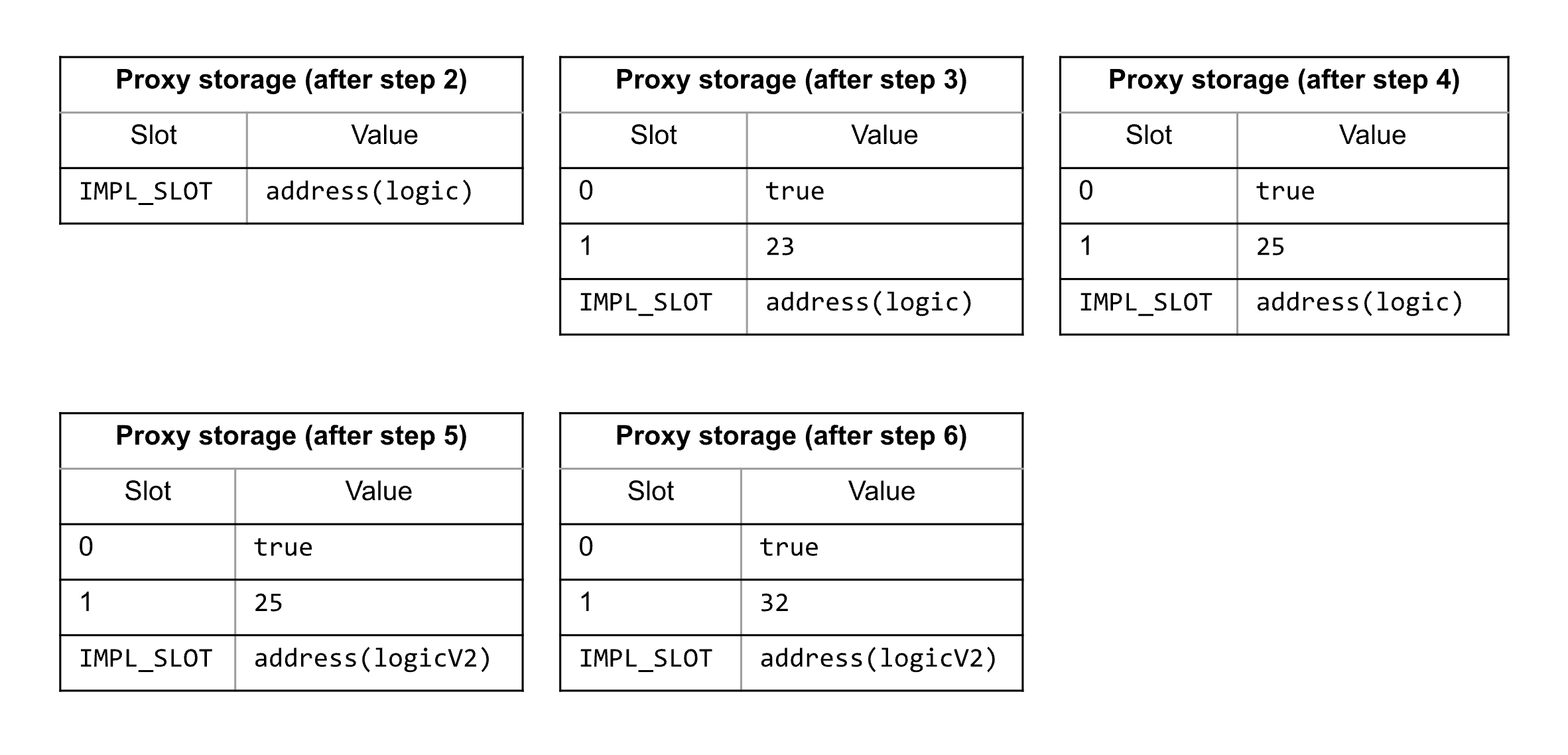When the proxy is created in step 2, it does not yet store any state from the logic contract. This only happens after step 3, when the logic contract is initialized, setting slot 0 (`isInitialized`) to `true` and slot 1 (`counter`) to 23.

See Proxy.t.sol for the full example.

## Design Choices

When implementing a proxy for upgradeable contracts, there are two fundamental questions that need to be answered:

1. How are storage slot collisions between proxy and logic contract prevented?
2. How are function selector clashes between proxy and logic contract dealt with?

In this post, we have only looked at the first question, and our answer was to use the Unstructured Storage Pattern. However, there are other approaches, like Inherited Storage or Eternal Storage (see Proxy Patterns for an overview).

Regarding the second question: As we have seen, functions are internally identified by function selectors that are four bytes long and derived from the keccak hash of the function signature. This makes it possible that functions with different signatures map to the same function selector, causing a selector clash.

For example, the selectors for the signatures `proxyOwner()` and `clash550254402()` are the same see here:

``````\$ cast keccak "proxyOwner()"
0x025313a28d329398d78fa09178ac78e400c933630f1766058a2d7e26bb05d8ea

\$ cast keccak "clash550254402()"
0x025313a2bba9fda619061d44004df81011846caa708c8d9abf09d256021e23ee
``````

Usually, this is not a problem, because if a function selector clash occurs between two functions of a single contract, then the Solidity compiler aborts with an error. However, if such a clash occurs between two functions from different contracts, then no error is reported because it normally does not matter. Except of course when using proxies: The fallback function of a proxy forwards any function to the logic contract that it does not define itself. Now, if the proxy and the logic contract define a function with the same selector, then the proxy will never forward calls to that function to the logic contract but will handle the call itself. See Malicious backdoors in Ethereum Proxies for more information.

There are at least two popular solutions to this problem: The Transparent Proxy Pattern and the Universal Upgradeable Proxy Standard (UUPS). The Transparent Proxy Pattern works by either forwarding all function calls to the logic contract or none at all, depending on the message sender. If the message sender is a designated proxy admin, then it is assumed that they only want to call functions on the proxy itself and never on the logic contract. For them, calls are never forwarded. On the other hand, for any other user it is assumed that they only want to call functions from the logic contract, and thus their calls are always forwarded. This avoids any problem stemming from function selector clashes, because the sender determines which contract should be used. For more information, see The Transparent Proxy Pattern.

The UUPS pattern is described in EIP-1822. Here, the problem of function selector clashes is avoided by simply not defining any public functions in the proxy. Instead, all the functionality for managing the proxy (including `upgradeTo()`) is implemented in the logic contract. See Transparent vs UUPS Proxies for more information.

As you may have noticed, our example Proxy contract implements neither the Transparent Proxy Pattern nor UUPS. In fact, it does not prevent function selector clashes at all and suffers from the exact problem described above. Have a look at the OpenZeppelin Proxy Library for production-ready proxy implementations.

Finally, there are other proxy patterns with different trade-offs. For example, there is the Beacon Proxy Pattern that introduces another level of indirection but allows to upgrade many contracts at once. There is also the Diamond Pattern that allows the logic to be spread out across multiple contracts, circumventing any code size restrictions.

## Conclusion and Outlook

In this post, we have developed a basic proxy implementation and discussed various pitfalls along the way. Still, our implementation has two major shortcomings:

1. It is vulnerable to function selector clashes
2. `upgradeTo()` is permissionless

We won’t address the first shortcoming in this series (see section Design Choices for potential solutions). However, in the next post, we will look more closely at the second one, because this will directly lead us to the vulnerability that was exploited in the Audius attack!

## Footnotes

1. Note that variables that occupy less than 32 bytes may be stored in the same slot. For example, a single slot can contain two variables of type `uint128`.

2. If we are talking in general about changing the behavior of a contract, then this is already possible with `selfdestruct` simply because it removes all code. While this is a very limited (and not very useful) form of changing a contract’s behavior, a lot more becomes possible when combined with create2. However, `delegatecall` remains the primary way to implement upgradeable contracts.

3. For simplicity, we revert with a fixed message instead of propagating the original error.

4. This differs from how arrays are laid out in storage, see Layout of State Variables in Storage and Layout in Memory.

5. The fallback function can also use a different signature where the calldata is directly passed as an argument. See the Solidity docs about fallback function for more information.

6. Why do we subtract one from the keccak hash? Purely from a functional point of view it makes no difference: Using the keccak hash without adding -1 works just as well. However, as mentioned in EIP-1967, the offset of -1 was added to make preimage attacks more difficult (also see https://github.com/ethereum/EIPs/pull/1967#issuecomment-489276813)